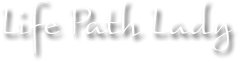### How to Calculate Numerology’s Universal and Personal Years

Jan
3

Hi everyone,

Here’s how you calculate the Universal Year:

Just take each number in the year and total them together:

For example, 2017 is:

2 + 0 + 1 + 7 = 10

Then you reduce that 10:

1 + 0 = 1

That means that 2017 is a #1 Year for the Universe.  This is the number that all of us share.  2017 is all about new beginnings.

Here’s how you calculate your Personal Year:

You take your birth month and day and add those numbers with the numbers of the current year.

For example, my birthday is August 4th:

8 + 4 +  2 + 0  + 1 + 7 = 22

Because #22 is a Master  Number, you don’t reduce it to a 4.

That means that 2017 is a #22 Year for me.  It’s all about my vision for the future.

Let’s do one more for practice:

If I had a client with a January 31st birthday:

1 + 3 + 1 + 2 + 0 + 1 + 7 = 15

1 + 5 = 6

2017 is a #6 personal year for her/him.  It’s all about family and home.